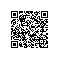# 不到 200 行代码，教你如何用 Keras 搭建生成对抗网络（GAN）## 判别器self.D = Sequential()
depth = 64
dropout = 0.4
# In: 28 x 28 x 1, depth = 1
# Out: 10 x 10 x 1, depth=64
input_shape = (self.img_rows, self.img_cols, self.channel)
activation=LeakyReLU(alpha=0.2)))
activation=LeakyReLU(alpha=0.2)))
activation=LeakyReLU(alpha=0.2)))
# Out: 1-dim probability
self.D.summary()

## 生成器self.G = Sequential()
dropout = 0.4
depth = 64+64+64+64
dim = 7
# In: 100
# Out: dim x dim x depth
# In: dim x dim x depth
# Out: 2*dim x 2*dim x depth/2
# Out: 28 x 28 x 1 grayscale image [0.0,1.0] per pix
self.G.summary()
return self.G

## 生成 GAN 模型

optimizer = RMSprop(lr=0.0008, clipvalue=1.0, decay=6e-8)
self.DM = Sequential()
self.DM.compile(loss='binary_crossentropy', optimizer=optimizer,\
metrics=['accuracy'])optimizer = RMSprop(lr=0.0004, clipvalue=1.0, decay=3e-8)
self.AM = Sequential()
self.AM.compile(loss='binary_crossentropy', optimizer=optimizer,\
metrics=['accuracy'])images_train = self.x_train[np.random.randint(0,
self.x_train.shape, size=batch_size), :, :, :]
noise = np.random.uniform(-1.0, 1.0, size=[batch_size, 100])
images_fake = self.generator.predict(noise)
x = np.concatenate((images_train, images_fake))
y = np.ones([2*batch_size, 1])
y[batch_size:, :] = 0
d_loss = self.discriminator.train_on_batch(x, y)
y = np.ones([batch_size, 1])
noise = np.random.uniform(-1.0, 1.0, size=[batch_size, 100])
a_loss = self.adversarial.train_on_batch(noise, y)

## 输出情况